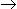# Chemical Engineering - Chemical Reaction Engineering

### Exercise :: Chemical Reaction Engineering - Section 5

11.

A catalyst in a chemical reaction

 A. decreases the activation energy. B. alters the reaction mechanism. C. increases the frequency of collisions of reacting species. D. all (a), (b) and (c).

Explanation:

No answer description available for this question. Let us discuss.

12.

Slurry reactors are characterised by the

 A. lack of intraparticle diffusion resistance. B. presence of two mobile phases. C. both (a) and (b). D. neither (a) nor (b).

Explanation:

No answer description available for this question. Let us discuss.

13.

Limiting reactant in a chemical reaction decides the

 A. rate constant B. conversion C. reaction speed D. equilibrium constant.

Explanation:

No answer description available for this question. Let us discuss.

14.

Consider the 'n' th order irreversible liquid phase reaction AB. Which one of the following plots involving half life of the reaction (t1/2) and the initial reactant concentration (CA0) gives a straight line plot ?

 A. CA0 Vs t1/2 B. ln CA0 Vs t1/2 C. CA0 Vs ln t1/2 D. ln CA0 Vs ln t1/2

Explanation:

No answer description available for this question. Let us discuss.

15.

The rate of forward reaction, at chemical equilibrium is____the rate of backward reaction.

 A. more than B. less than C. equal to D. either (b) or (c)